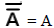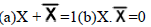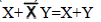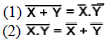Tuesday 26th October 2021
 CBSE Computer Science - Revision Tour(Solved) CBSE Guess > eBooks > Class XII > CBSE Computer Science Boolean Algebra Laws Solved Revision Tour By Mr. Ravi Kiran COMPUTER SCIENCE BOOLEAN ALGEBRA LAWS Properties of 0 and 1: 0 + X = X, 1 + X = 1, 0.X = 0, 1.X = X Idempotence Law: (a) X +X = X (b) X.X = X Involution Law:ComplementaryLaw:Commutative Law: (a) X+Y = Y+X (b) X.Y = Y.X Associative Law: (a) X + (Y+Z) = (X+Y) + Z (b) X.(Y.Z) = (X.Y).Z Distributive Law: (a) X(Y+Z) = XY + XZ (b) X+YZ = (X+Y) (X+Z) Absorption Law: (a) X + XY = X (b) X (X+Y) + X Third distributive Law:Demorgan’s TheoremsCBSE Computer Science Solved Revision Tour By Mr. Ravi Kiran ( [email protected] )